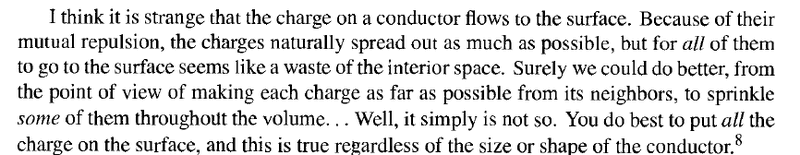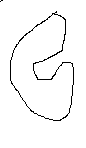# Charge on conductors

• person_random_normal

#### person_random_normal- David J Griffiths

But how s it valid for some conductor like →Given:
1) Maxwells equations.
2) Electrostatic: ##J=0##, ##d\rho/dt=0##, ##dE/dt=0##, ##dB/dt=0##
3) Conductor: ##J=\sigma E##

Assume there is charge in the interior. Then by Gauss law there is an E field in the interior. Then by assumption 3) there is a current. This contradicts assumption 2). Therefore there can be no charge in the interior.

So does that mean -
if some charge is given to an arbitrarily shaped conductor, it will surface and regardless of everything; the field inside will be zero and the surface will be equipotential

somebody !

The reasoning is very simple. I don't know, why Griffiths likes to obscure things in his textbooks. In electrostatics by definition we have all fields and sources time-independent and also no current density (see #3). Then within a conductor you have
$$\sigma \vec{E}=\vec{j}=0 \; \Rightarrow \; \vec{E}=0.$$
The electric charge density at every point is given by Gauss's Law (Heaviside-Lorentz units)
$$\vec{\nabla} \cdot \vec{E}=\rho.$$
Since ##\vec{E}=0## inside the conductor also ##\rho=\vec{\nabla} \cdot \vec{E}=0## inside the conductor. So if the conducting body is overall charged, the charge must be on its surface.

Caveat: To be more accurate, of course microscopically the entire body consists of charged particles (atomic nuclei and electrons). So there are charges inside the body but they are compensating each other precisely in the static case, when looked from a macroscopic (coarse-grained) point of view. So within macroscopic electrodynamics the macroscopic charge distribution vanishes in conductors for electrostatic situations.

so #4 is right ?

So does that mean -
if some charge is given to an arbitrarily shaped conductor, it will surface and regardless of everything; the field inside will be zero and the surface will be equipotential

The electric charge density at every point is given by Gauss's Law (Heaviside-Lorentz units)
⃗∇⋅⃗E=ρ.​
by the way can you put this physically , I don't know this math

So does that mean -
if some charge is given to an arbitrarily shaped conductor, it will surface and regardless of everything; the field inside will be zero and the surface will be equipotential
Only in the electrostatic case, yes. This does not hold in magnetostatics or electrodynamics.

•person_random_normal
So does that mean -
if some charge is given to an arbitrarily shaped conductor, it will surface and regardless of everything; the field inside will be zero and the surface will be equipotential
Yes. The surface is equipotential.

That does not mean that the surface charge is uniformly distributed.

Yes. The surface is equipotential.

That does not mean that the surface charge is uniformly distributed.

Then we should have current over the surface, as its made of conductor, does that happen in steady state ?
It won't be electrostatics then !

Then we should have current over the surface, as its made of conductor, does that happen in steady state ?
It won't be electrostatics then !
I didn't mention current, nor did I imply it.

The surface charge density is not one uniform value over the whole surface. It varies from location to location, but it is static.

I didn't mention current, nor did I imply it.

The surface charge density is not one uniform value over the whole surface. It varies from location to location, but it is static.

that means it is equipotential

that means it is equipotential
Yes.